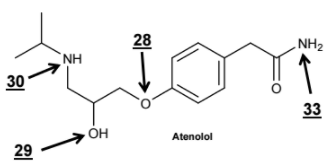# Problem: Look at the  entire structure of Atenolol. What is the  total number of lone pairs present within the structure of this molecule? A. 0 B. 1 C. 2 D. 3 E. 4 F. 5 G. 6 H. 7 I.  8 J. None of these

###### FREE Expert Solution
90% (477 ratings)
###### Problem Details

Look at the  entire structure of Atenolol. What is the  total number of lone pairs present within the structure of this molecule?

A. 0

B. 1

C. 2

D. 3

E. 4

F. 5

G. 6

H. 7

I.  8

J. None of theseWhat scientific concept do you need to know in order to solve this problem?

Our tutors have indicated that to solve this problem you will need to apply the Introduction to Organic Chemistry concept. You can view video lessons to learn Introduction to Organic Chemistry. Or if you need more Introduction to Organic Chemistry practice, you can also practice Introduction to Organic Chemistry practice problems.

What is the difficulty of this problem?

Our tutors rated the difficulty ofLook at the  entire structure of Atenolol. What is the  tota...as high difficulty.

How long does this problem take to solve?

Our expert Chemistry tutor, Dasha took 2 minutes and 57 seconds to solve this problem. You can follow their steps in the video explanation above.

What professor is this problem relevant for?

Based on our data, we think this problem is relevant for Professor Staff's class at USF.